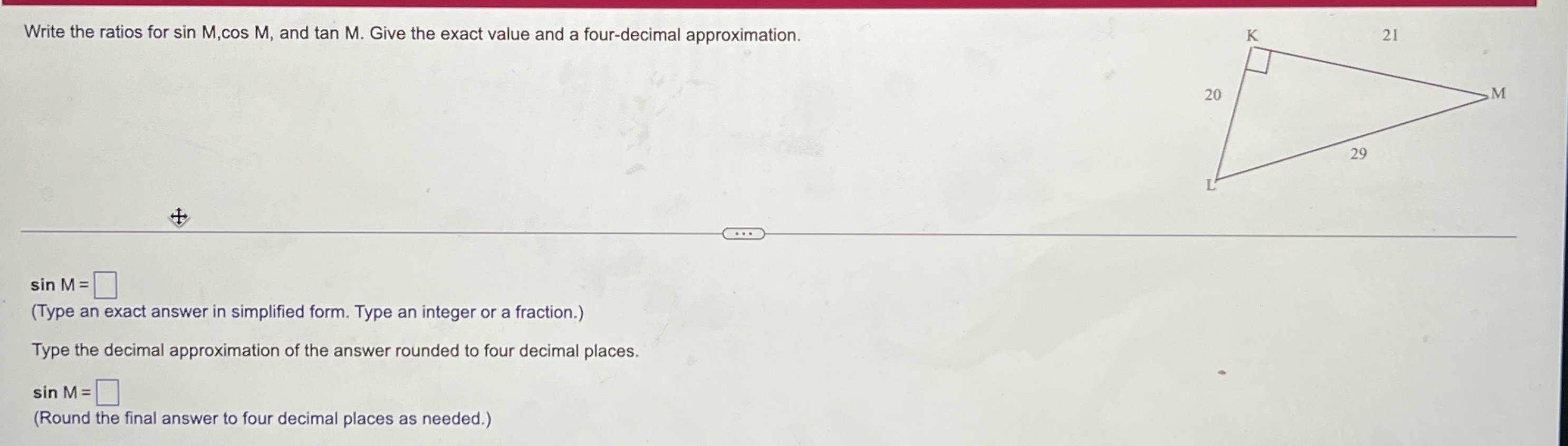### Still have math questions?

Trigonometry
QuestionWrite the ratios for $$\sin M , \cos M ,$$ and $$\tan M$$ . Give the exact value and a four-decimal approximation.

$$\sin M = \square$$

(Type an exact answer in simplified form. Type an integer or a fraction.) Type the decimal approximation of the answer rounded to four decimal places.

$$\sin M = \square$$

(Round the final answer to four decimal places as needed.)